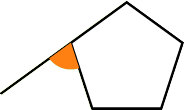There’s a simple rule governing what are known as the ‘exterior angles’ of a polygon. If you take any of the angles of a polygon, no matter how many sides, and extend a line from one of the two sides that meet at that point, you create two angles where there was previously only one. Since you’ve only lengthened a straight line, the two angles must add up to 180°. The one outside the polygon is called the ‘exterior angle’, compared to the ‘interior angle, which is the one inside the polygon.The reason this is interesting is that no matter how may sides that polygon has, if you add up the exterior angles they will come to 360° - always.

If you want to work that out for yourself, it’s not hard. Take any polygon and break it up into triangles, all of which meet at one of the vertices (corners) like this:  You will always end up with a number of triangles which is equal to the number of sides minus two. Since the angles inside a triangle add up to 180, the total of all the angles is 180 * (number of sides – 2).Now look at the exterior angles above. Each exterior angle shares a straight line with one of the interior angles, so together they add up to 180° and there is one for each corner/side. So if we take away the sum of all the internal angles from the sum of all those exterior + interior angles we have (number of sides)*180° - (number of sides – 2)*180°, which leaves 2*180° = 360°.

But there is a neat visual demonstration of the same fact, which is represented by this design. If you create all the exterior angles and then shrink the polygon until ultimately it disappears completely, leaving only the exterior angles, they smoothly merge to form a circle, or 360°.

The statements about the sum of the internal angles and the exterior angles works for any polygon, but only regular polygons – those with equal sides – produce the simple  ‘shrink to a circle’ effectI call this one simply '360'.